# C. Consider the dissolution of CaF2 in water. The Ksp of CaF2 is 1.5 x 1010 at 25°C Qualitatively...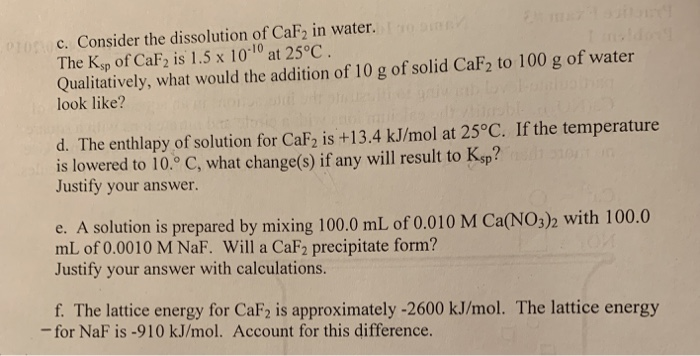c. Consider the dissolution of CaF2 in water. The Ksp of CaF2 is 1.5 x 1010 at 25°C Qualitatively, what would the addition of 10 g of solid CaF2 to 100 g of water look like? d. The enthlapy of solution for CaF2 is +13.4 kJ/mol at 25°C. If the temperature is lowered to 10° C, what change(s) if any will result to Ksp? Justify your answer. e. A solution is prepared mL of 0.0010 M NaF. Will a CaF2 precipitate form? Justify your answer with calculations. red by mixing 1000 ml. oro.oio M CaNOs)h with 100.0 f. The lattice energy for CaF2 is approximately -2600 kJ/mol. The lattice energy -for NaF is -910 kJ/mol. Account for this difference.

c. Density of water=1 g/mL

So volume of 100 g water=mass/density=100 g/1 g/mL=100 mL=100 mL/1000 mL/L=0.1 L (1000 mL=1 L)

Molar mass of CaF2=Molar mass of Ca+2xmolar mass of F=40 g/mol+2x19 g/mol=40 g/mol+38 g/mol=78 g/mol

Number of moles of CaF2=mass/molar mass=10 g/78 g/mol=0.13 mol

Molarity of CaF2=number of moles/volume of solution (L)

=0.13 mol/0.1 L=0.013 M

CaF2 dissociates as shown in the solution0.013 M 2x0.013 M

=0.026 M

Reaction quotient Q==0.013 M x (0.026 M)2=8.8x10-6 M3

As Q>Ksp so CaF2 will precipitate out. Qualitatively, 10 g Calcium fluoride added to 100 g water doesn't dissolve completely.

d. Since enthalpy of dissolution is positive, it means it is an endothermic reaction. On lowering the temperature of an endothermic reaction it moves in a direction so as to increase the temperature i.e. in backward direction. So lowering the temperature reduces the solubility of calcium fluoride and hence lowers its Ksp.

e. Number of moles of calcium nitrate=Molarity x Volume

=0.010 M x 100 mL/1000 mL/L

=0.001 mol

Concentration of calcium nitrate in diluted solution=number of moles/volume of the solution=0.001 mol/200 mL/1000 mL/L=0.005 M

1 molecule of CaF2 gives 1 Ca+2 in solution

So concentration of Ca+2 in solution=0.005 M

Number of moles of sodium fluoride=Molarity x Volume

=0.0010 M x 100 mL/1000 mL/L

=0.0001 mol

Concentration of NaF in diluted solution=number of moles/Volume (L)

=0.0001 mol/200 mL/1000 mL/L=0.0005 M

1 molecule of NaF gives 1 F- in solution

So concentration of F- in diluted solution=0.0005 M

Reaction quotient Q==0.005 M x (0.0005 M)2=1.25x10-9 M3

As Q>Ksp, a precipitate of calcium fluoride will form.

f. In CaF2, we have ionic bonding between Ca+2 (bivalent cation) and F-​​​. In NaF the ionic bonding is between Na+ (monovalent cation) and F-. So the electrostatic forces of attraction is more in CaF2 than in NaF due to higher charge on calcium ion than sodium ion. So lattice energy is higher for calcium fluoride than NaF.

##### Add Answer of: C. Consider the dissolution of CaF2 in water. The Ksp of CaF2 is 1.5 x 1010 at 25°C Qualitatively...
Similar Homework Help Questions
• ### MI Review | Constants 1 Periodi Consider the dissolution of AgBr in water at 25 'C:...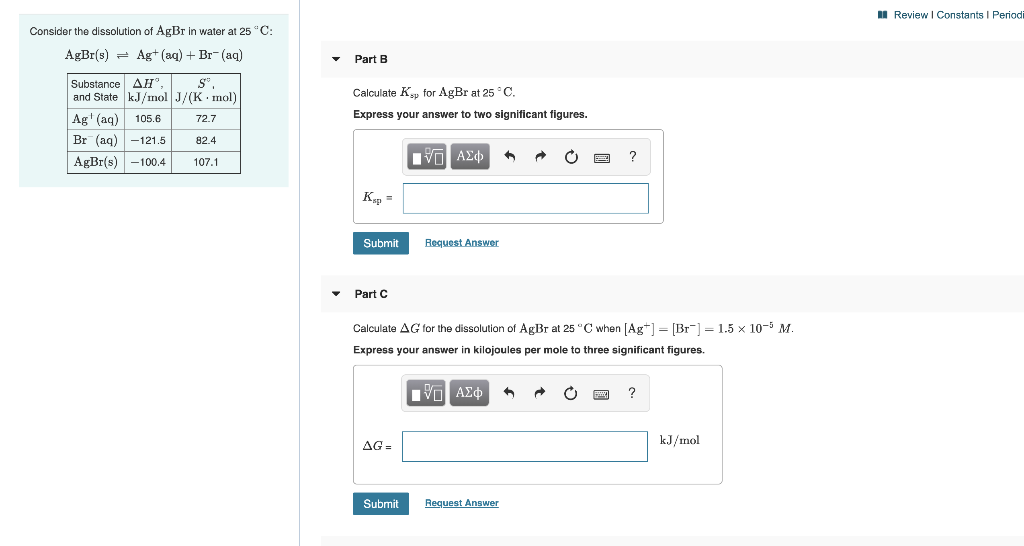MI Review | Constants 1 Periodi Consider the dissolution of AgBr in water at 25 'C: AgBr(s) = Ag+ (aq) + Br-(aq) Part B Substance AH' S and State kJ/mol J/(K.mol) Ag' (aq) 105.6 72.7 Br (aq) –121.5 82.4 AgBr(s) -100.4 107.1 Calculate Ksp for AgBr at 25°C. Express your answer to two significant figures. VA ALQ O 2 ? KE Submit Request Answer Part C Calculate AG for the dissolution of AgBr at 25°C when [Ag+] = [Br") –...

• ### Sodium hydroxide (NaOH) has a lattice energy of -887 kJ/mol and a heat of hydration of -932...

Sodium hydroxide (NaOH) has a lattice energy of -887 kJ/mol and a heat of hydration of -932 kJ/mol.How much solution could be heated to boiling by the heat evolved by the dissolution of 24.5 g of NaOH? (For the solution, assume a heat capacity of 4.0 J/g C, aninitial temperature of 25.0 C, a boiling point of 100.0 C, and a density of 1.05 g/mL.)V= ? mLPlease show work. :)

• ### Calculate the solubility at 25 °C of CaF2 in pure water and in a 0.0060 M NaF solution. You'll find K. data in the...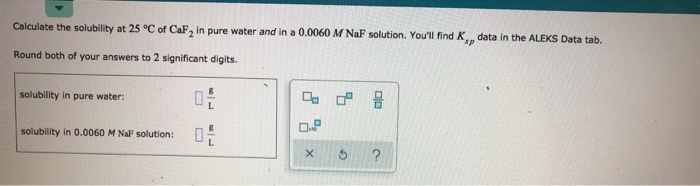Calculate the solubility at 25 °C of CaF2 in pure water and in a 0.0060 M NaF solution. You'll find K. data in the ALEKS Data tab. Round both of your answers to 2 significant digits. solubility in pure water: solubility in 0.0060 M Naf solution: x 6 ?

• ### Question 29 of 50 Submit 5.21 g of MgSO4 is placed into 100.0 mL of water....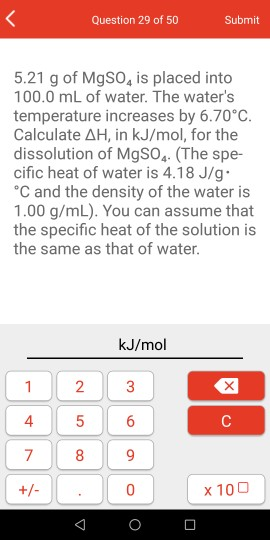Question 29 of 50 Submit 5.21 g of MgSO4 is placed into 100.0 mL of water. The water's temperature increases by 6.70°C. Calculate AH, in kJ/mol, for the dissolution of MgSO4 (The spe- cific heat of water is 4.18 J/g. *C and the density of the water is 1.00 g/mL). You can assume that the specific heat of the solution is the same as that of water. kJ/mol 1 2 3 C +/- : 0 x 100

• ### What is the final temperature of the solution when 4.806 g of sodium hydroxide is dissolved in 100.0 mL of water at an i...

What is the final temperature of the solution when 4.806 g of sodium hydroxide is dissolved in 100.0 mL of water at an initial temperature of 24.5 C?. The density of water is 0.9969 g/cm3, and the heat capacity is 4.184 J/C. The enthalpy of dissolution for sodium hydroxide is -44.2 kJ/mol.

• ### For CaF2 Kap = 3.9 x 10-" (molar mass = 78 g mol-') at 25°C. How...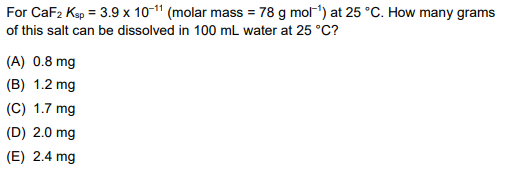For CaF2 Kap = 3.9 x 10-" (molar mass = 78 g mol-') at 25°C. How many grams of this salt can be dissolved in 100 mL water at 25°C? (A) 0.8 mg (B) 1.2 mg (C) 1.7 mg (D) 2.0 mg (E) 2.4 mg

• ### For full marks in the following questions, you must provide the Ksp algebraic expression and all...

For full marks in the following questions, you must provide the Ksp algebraic expression and all relevant chemical equations including source equations. The solubility of Ag2CO3 in water is 0.038 g / L. What is the Ksp of Ag2CO3? The Ksp of Pb(IO3)2 is 3.7 x 10-12 at 25 0C. What is the solubility of Pb(IO3)2 in water in g / L at 250 C? 200 ml of a saturated solution of BaF2 was evaporated to dryness and yielded 1.24...

• ### When a solid dissolves in water, heat may be evolved or absorbed. The heat of dissolution (dissolving) can be det...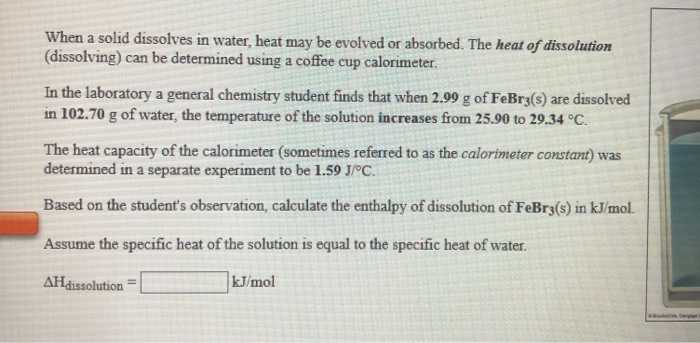When a solid dissolves in water, heat may be evolved or absorbed. The heat of dissolution (dissolving) can be determined using a coffee cup calorimeter. In the laboratory a general chemistry student finds that when 2.99 g of FeBrz(s) are dissolved in 102.70 g of water, the temperature of the solution increases from 25.90 to 29.34 °C. The heat capacity of the calorimeter (sometimes referred to as the calorimeter constant) was determined in a separate experiment to be 1.59 J/°C....

• ### ksp is 3.45x10-11 Calculate the solubility at 25 °C of CaF, in pure water and in a 0.0110 MNaF solution. Round both...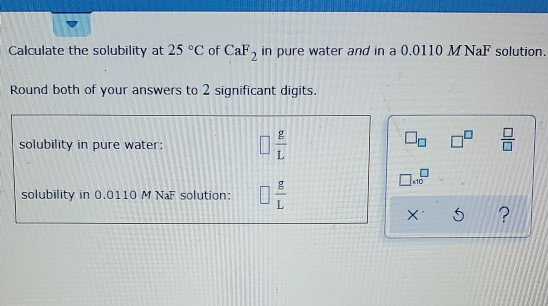ksp is 3.45x10-11 Calculate the solubility at 25 °C of CaF, in pure water and in a 0.0110 MNaF solution. Round both of your answers to 2 significant digits. solubility in pure water: L solubility in 0.0110 M NaF solution: ? X

• ### The solubility of Ag2CrO4 at 25 °C in water is 0.0027 g/100 mL. Estimate Ksp for...

The solubility of Ag2CrO4 at 25 °C in water is 0.0027 g/100 mL. Estimate Ksp for this substance?

Need Online Homework Help?